Question

(a) Use Mathematica to find an echelon form of A. (b) Using your answer to the...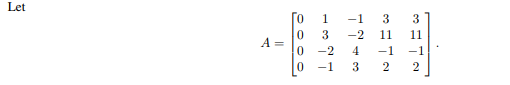(a) Use Mathematica to find an echelon form of A.

(b) Using your answer to the previous part, find the rank and nullity of A.

(c) Find a basis for the row space of A.

(d) Find a basis for the column space of A.

(e) Find a basis for the null space of A.

Let To 1 3 3 0 -1 3 2 2

(a) solution: reduced row space echelon form of A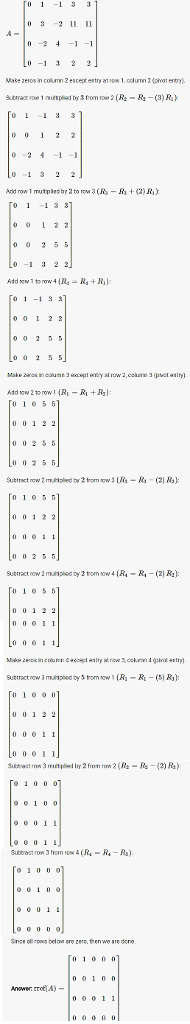(b) rank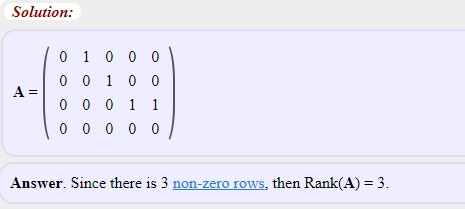nullity:

rankA + nullityA = number of columns of A.

therefore, nullityA= no. of columnsA - rankA = 5-3 =2

(c) row space of A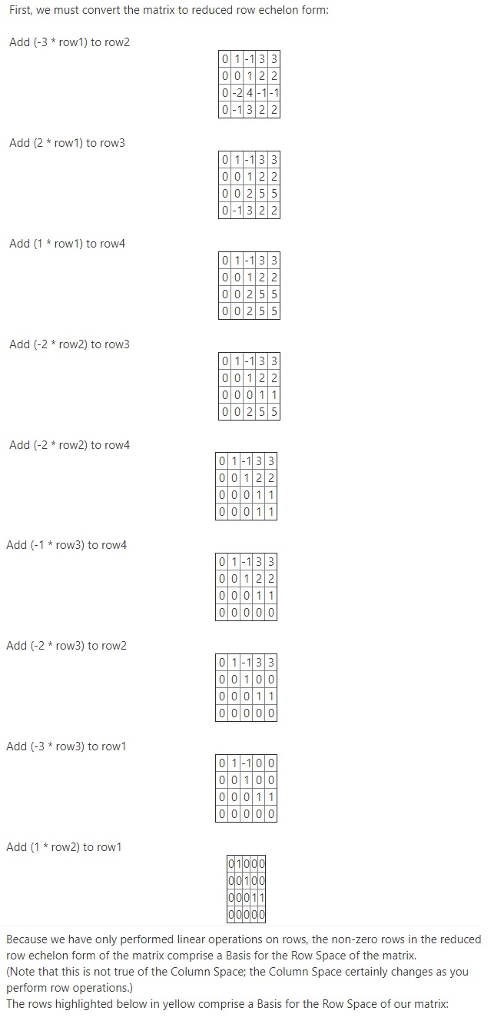(d) column space of A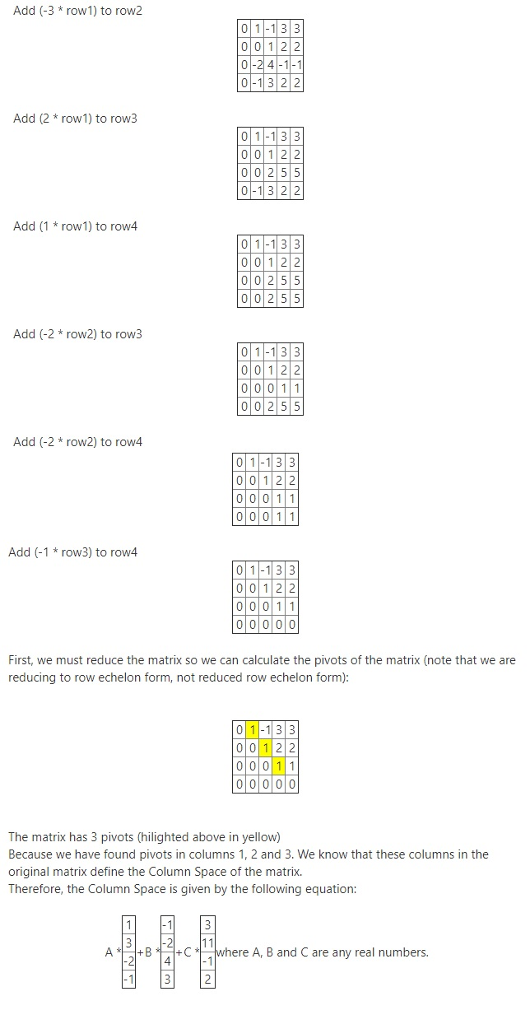(e) null space of A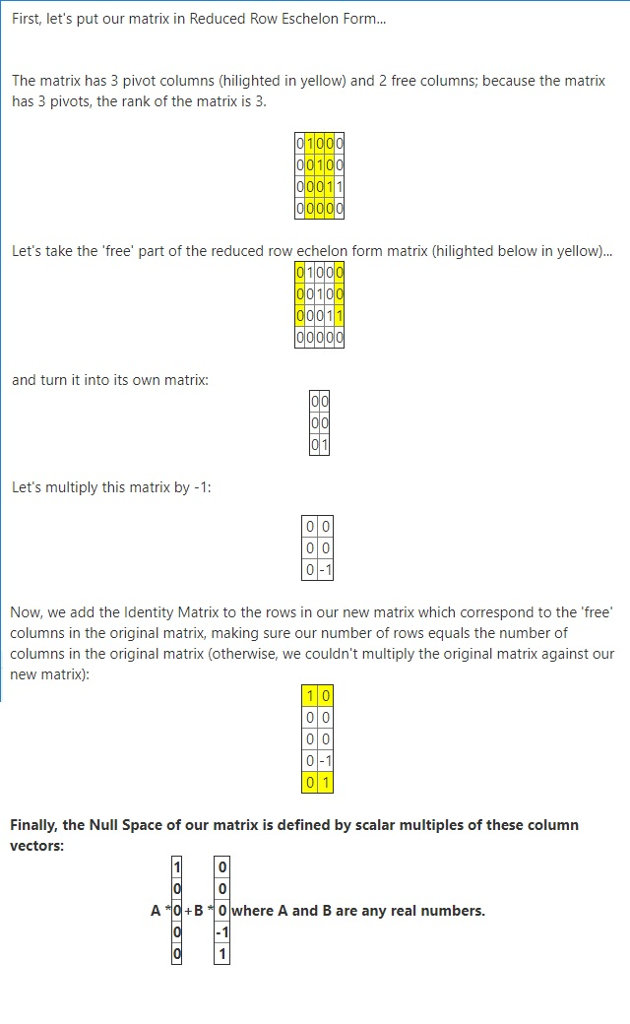Earn Coins

Coins can be redeemed for fabulous gifts.

Similar Homework Help Questions
• Given the matrix A and its reduced row echelon form R, answer the following questions. A=...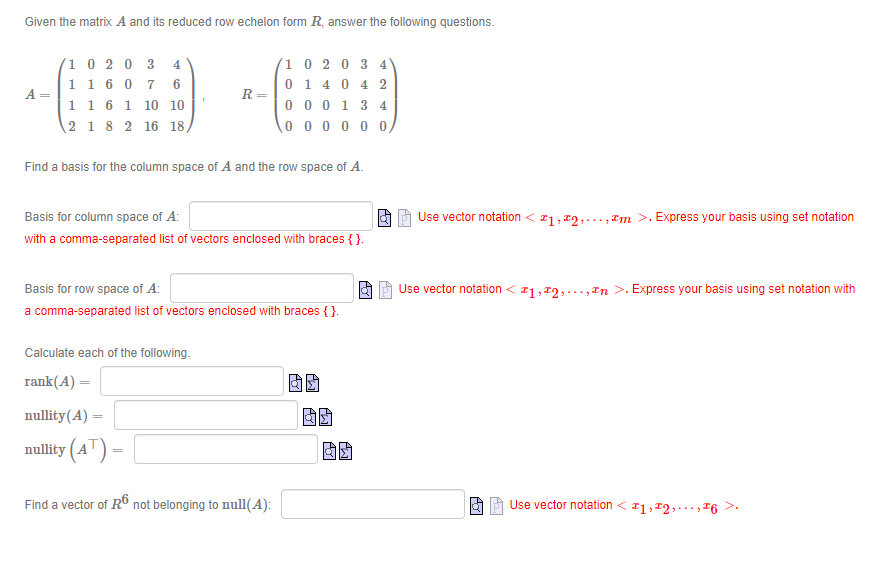Given the matrix A and its reduced row echelon form R, answer the following questions. A= 10 20 3 4 1 1 60 7 6 11 6 1 10 10 2 1 8 2 16 18 R= 1 0 2 0 3 4 0 14 0 4 2 000134 000000 Find a basis for the column space of A and the row space of A. Basis for column space of A: with a comma-separated list of vectors enclosed with braces...

• Given the matrix A and its reduced row echelon form R, answer the following questions. 4...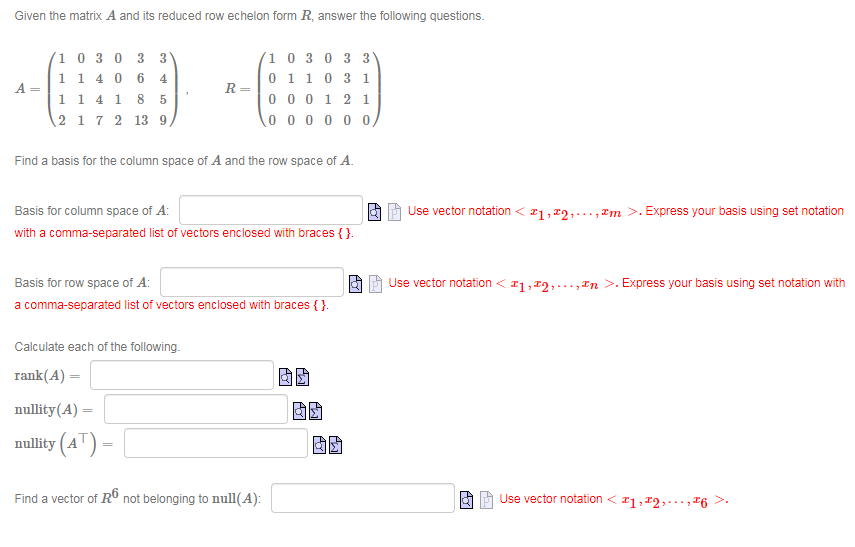Given the matrix A and its reduced row echelon form R, answer the following questions. 4 A 1 0 3 0 3 3 1 1406 1 1 4 1 8 2 1 7 2 13 9 R= 1 0 3 0 3 3 0110 31 000121 1000000 5 Find a basis for the column space of A and the row space of A. Ao Basis for column space of A: with a comma-separated list of vectors enclosed with braces {}....

• Given the matrix A and its reduced row echelon form R, answer the following questions. A=...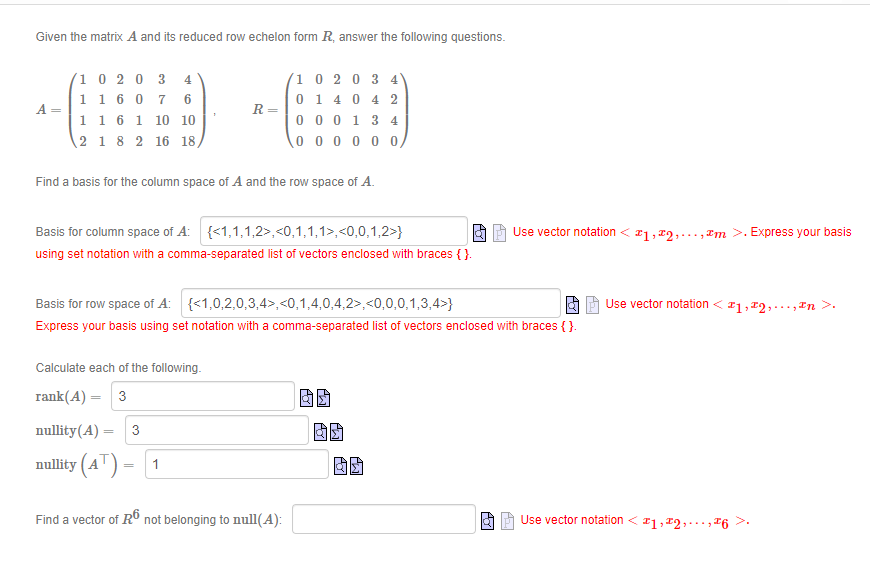Given the matrix A and its reduced row echelon form R, answer the following questions. A= 1 02 03 4 1 1 6 0 7 6 1 1 6 1 10 10 2 1 8 2 16 18 R= (10 20 3 4 01 4 0 4 2 000134 000000 Find a basis for the column space of A and the row space of A. Use vector notation <21,22,..., Im >. Express your basis Basis for column space of A:...

• Question 3 please answer clearly. A matrix A and its reduced row echelon form are given...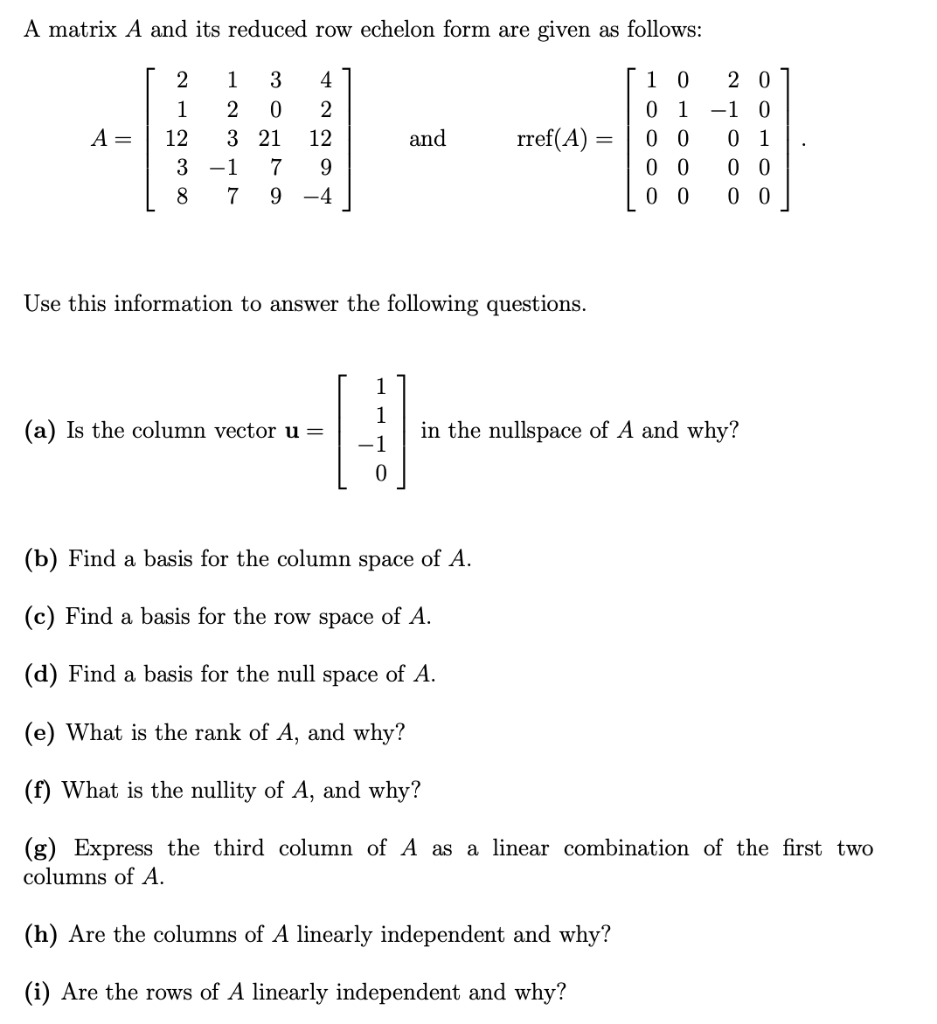Question 3 please answer clearly. A matrix A and its reduced row echelon form are given as follows: [ 2 1 3 41 | 1 2 0 2 A= 3 21 12 | 3 -1 7 9 18 7 9 -4 and rref(A) = [ 1 0 201 0 1 -1 0 0 0 0 1 0 0 0 0 | 0 0 0 0 Use this information to answer the following questions. (a) Is the column vector u= in...

• And its row echelon form (verify ) is given by 1-3 4-2 5 0 01 3- what is the nullity of A without...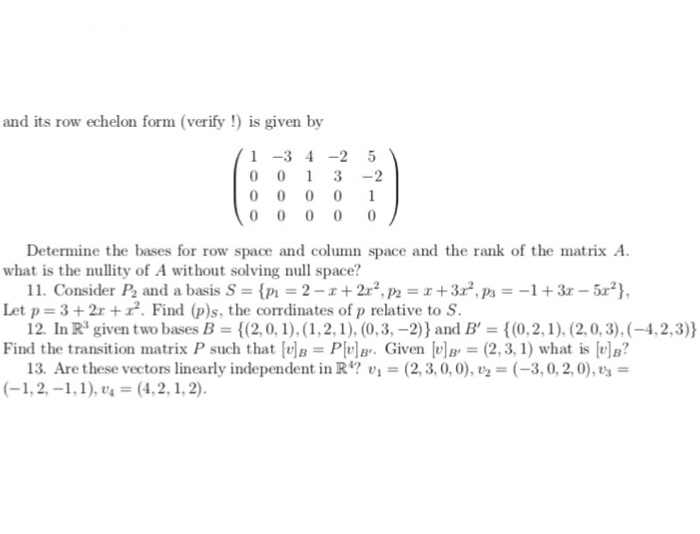Need answer 11~13，as detailed as possible please and its row echelon form (verify ) is given by 1-3 4-2 5 0 01 3- what is the nullity of A without solving null space? Let p 3+2r+. Find (p)s, the corrdinates of p relative to S. Find the transition matrix P such that [tle = Plula.. Given lula, = (2,3, 1) what is lul? Determine the bases for row space and column space and the rank of the matrix A 11....

• [1 -1 0 0 -2 0] 1 4 -4 0 0 -8 0 (1 point) Let...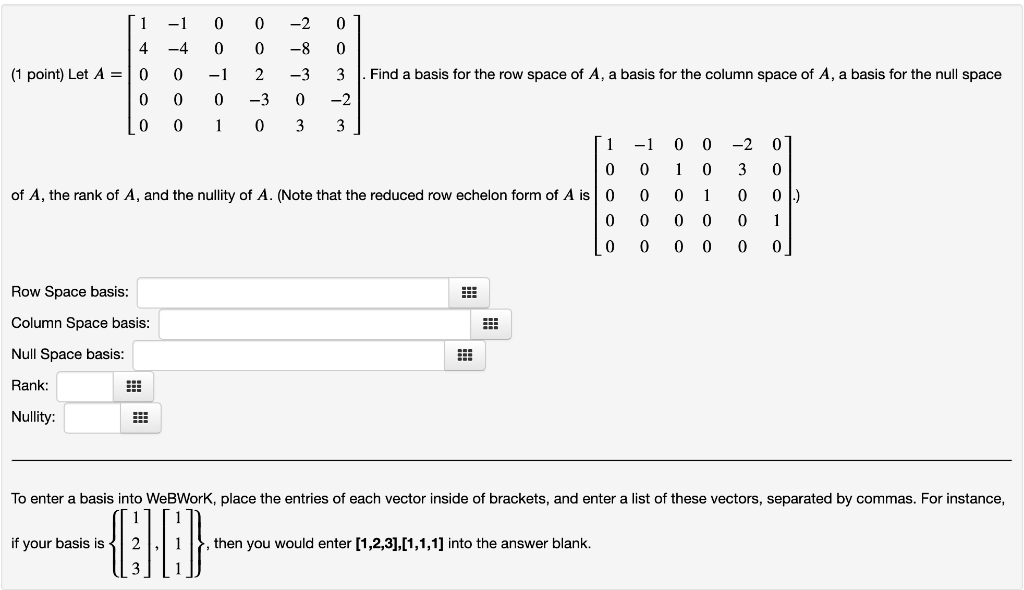[1 -1 0 0 -2 0] 1 4 -4 0 0 -8 0 (1 point) Let A = 10 0 -1 2 -3 3 . Find a basis for the row space of A, a basis for the column space of A, a basis for the null space 0 0 0 -3 0 -2 Lo 0 1 0 3 3] [1 -1 0 0 -2 01 0 0 1 0 3 0 of A, the rank of A, and the...

• 1 1. The matrix A and it reduced echelon form B are given below. 1 -2...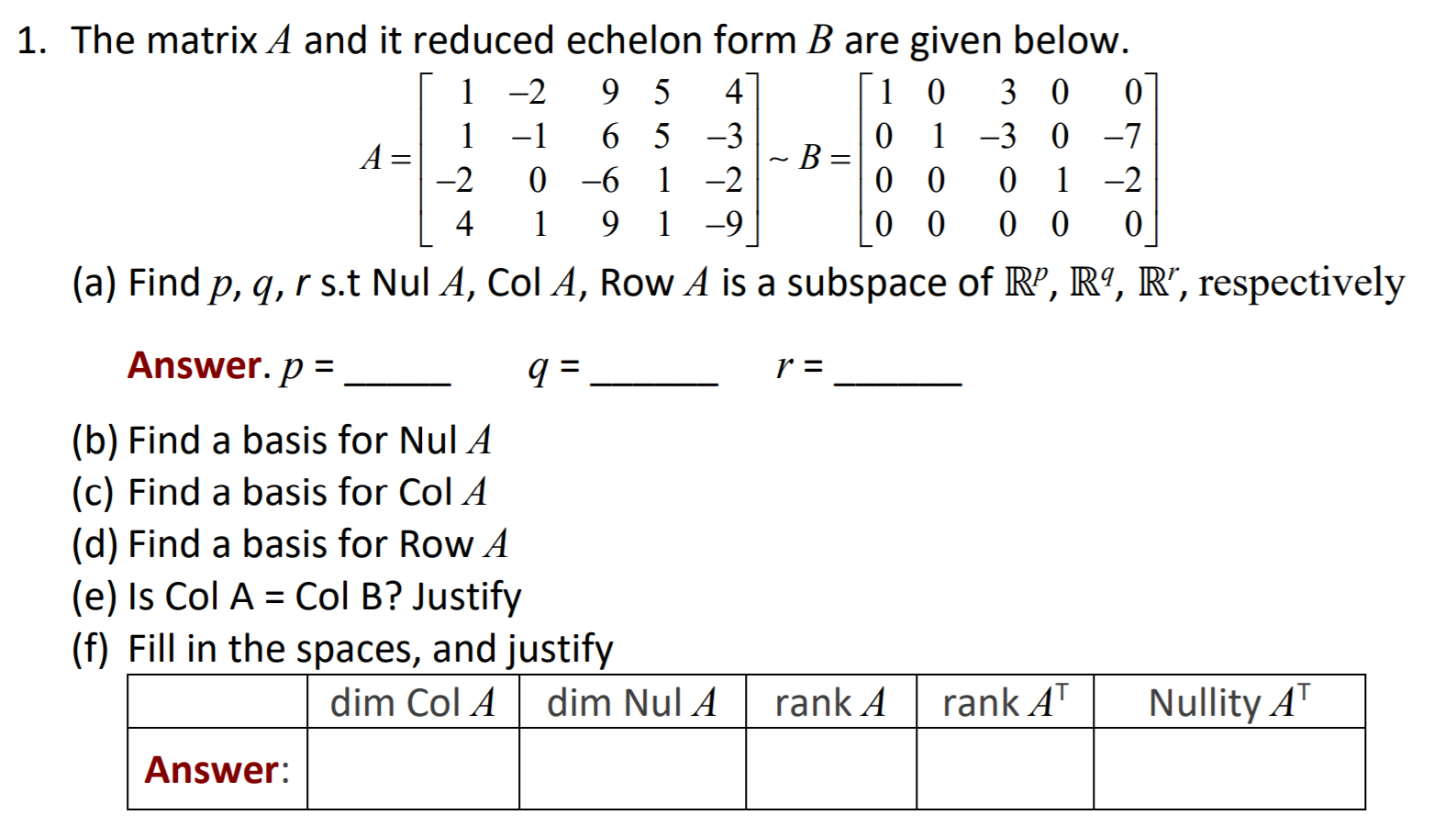1 1. The matrix A and it reduced echelon form B are given below. 1 -2 9 5 4 1 0 3 0 0 -1 6 5 -3 0 1 -3 0 -7 A= ~B= -2 0 -6 1 -2 0 0 1 -2 4 9 1 -9 0 0 0 0 0 (a) Find p, q, r s.t Nul A, Col A, Row A is a subspace of RP, R9, R”, respectively o 1 Answer. p = a =...

• 4 1 1. The matrix A and it reduced echelon form B are given below. 1-2...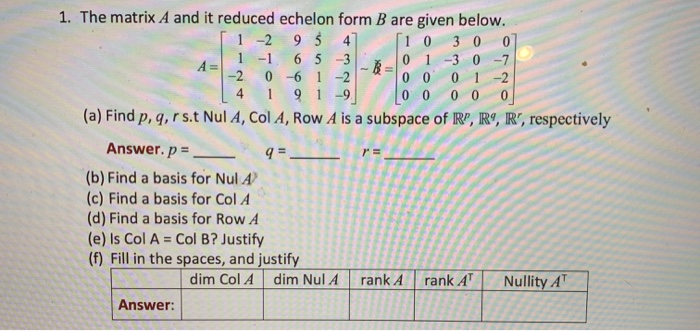4 1 1. The matrix A and it reduced echelon form B are given below. 1-2 95 4 10 3 0 0 1 -1 6 5 3 0 1 -3 0 -7 -2 0 -6 1 -2 0 0 0 1 -2 91-9 0 0 0 0 0 (a) Find p, q, rs. Nul A, Col A, Row A is a subspace of R", R9, R', respectively Answer. p = 9=- (b) Find a basis for Nul A (c) Find...

• -2 1 2. (12 pts) Given the matrix in a R R-E form: [1 0 0...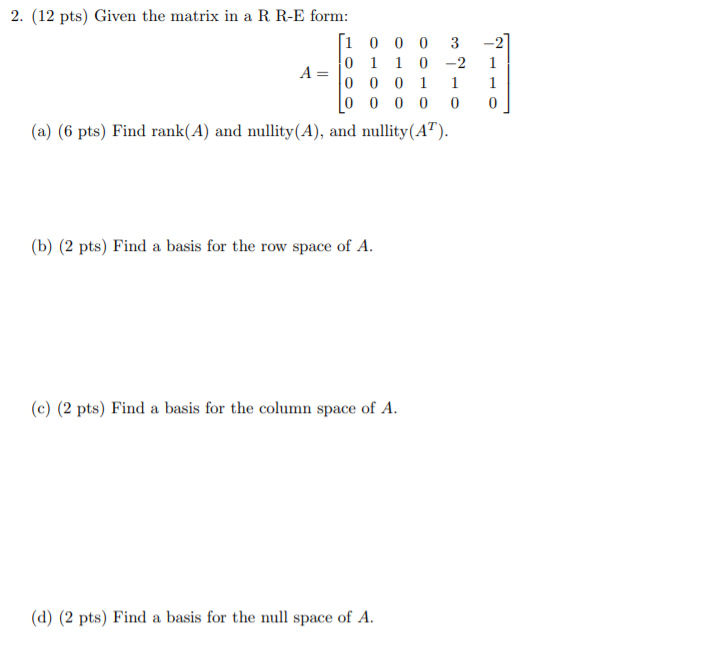-2 1 2. (12 pts) Given the matrix in a R R-E form: [1 0 0 0 3 0 1 1 0 -2 A 0 0 0 1 [0 0 0 0 0 (a) (6 pts) Find rank(A) and nullity(A), and nullity (AT). 1 0 (b) (2 pts) Find a basis for the row space of A. (c) (2 pts) Find a basis for the column space of A. (d) (2 pts) Find a basis for the null space of...

• no calculator please 2. (12 pts) Given the matrix in a R R-E form: [1 0...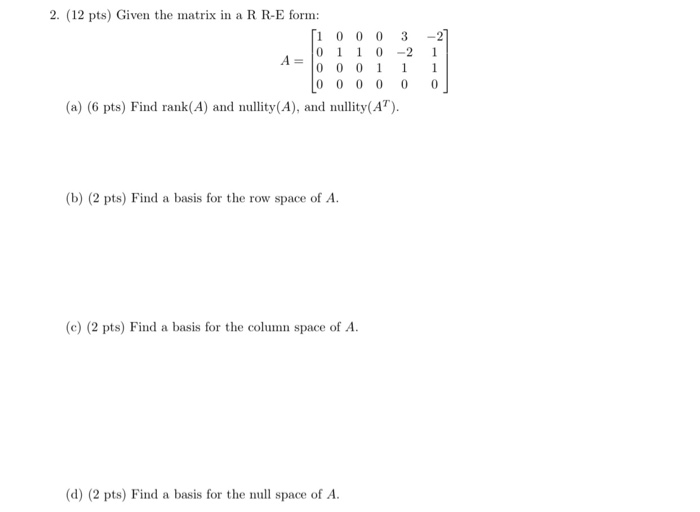no calculator please 2. (12 pts) Given the matrix in a R R-E form: [1 0 0 0 3 -2 1 1 0 0 0 0 0 1 0 0 0 0 0 0 (a) (6 pts) Find rank(A) and nullity(A), and nullity(AT). (b) (2 pts) Find a basis for the row space of A. (c) (2 pts) Find a basis for the column space of A. (d) (2 pts) Find a basis for the null space of A.